# Basic Features in WinUI Slider

30 Jun 20217 minutes to read

This section explains about how to add the WinUI slider with basic features.

## Setting Minimum and Maximum value

The `Minimum` and `Maximum` properties of a Slider is used to customize the start and end range of the Slider. The default value of `Minimum` is 0 and `Maximum` is 100.

``````<slider:SfSlider Minimum="-20"
Maximum="20"
ShowLabels="True" />``````
``````SfSlider sfSlider = new SfSlider();
sfSlider.Minimum = -20;
sfSlider.Maximum = 20;
sfSlider.ShowLabels = true;
this.Content = sfSlider;``````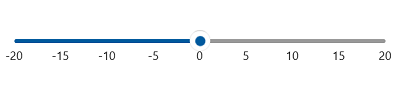## Interval

Slider elements like labels, ticks, and dividers are rendered based on the `Interval`, `Minimum`, and `Maximum` properties. The default value of `Interval` is double.NaN.

For example, if `Minimum` is 0, `Maximum` is 10, and `Interval` is 2, the slider will render the labels, major ticks, and dividers at 0, 2, 4 and so on.

``````<slider:SfSlider Minimum="0"
Maximum="10"
Interval="2"
Value="4"
ShowTicks="True"
ShowLabels="True" />``````
``````SfSlider sfSlider = new SfSlider();
sfSlider.Minimum = 0;
sfSlider.Maximum = 10;
sfSlider.Interval = 2;
sfSlider.Value = 4;
sfSlider.ShowTicks = true;
sfSlider.ShowLabels = true;
this.Content = sfSlider;``````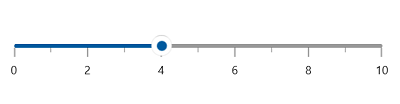NOTE

Slider having auto-interval support. So, the auto-interval is calculated by default.

NOTE

## Discrete Selection for Values

You can move the thumb in discrete manner for numeric values using the `StepFrequency` property in the range slider.

``````<slider:SfSlider Minimum="0"
Maximum="10"
Interval="2"
Value="4"
StepFrequency="2"
ShowTicks="True"
ShowLabels="True" />``````
``````SfSlider sfSlider = new SfSlider();
sfSlider.Minimum = 0;
sfSlider.Maximum = 10;
sfSlider.Interval = 2;
sfSlider.Value = 2;
sfSlider.StepFrequency = 2;
sfSlider.ShowTicks = true;
sfSlider.ShowLabels = true;
this.Content = sfSlider;``````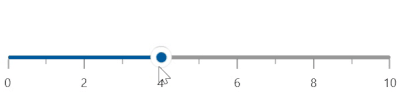## Value

You can show value in the slider by setting double value to the `Value` properties.

``````<slider:SfSlider Value="50"
ShowLabels="True" />``````
``````SfSlider sfSlider = new SfSlider();
sfSlider.Value = 50;
sfSlider.ShowLabels = true;
this.Content = sfSlider;``````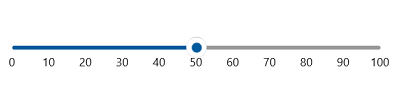## Flow Direction Customization

The direction of slider can be customized by its `IsInversed` property.

When the `IsInversed` property is true, the slider can be placed in right-to-left direction. When the `IsInversed` property is set to false, the slider will be positioned in left-to-right direction.

``````<slider:SfSlider ShowTicks="True"
ShowLabels="True"
Interval="20"
Value="40"
IsInversed="True"/>``````
``````SfSlider sfSlider = new SfSlider();
sfSlider.ShowTicks = true;
sfSlider.ShowLabels = true;
sfSlider.Interval = 20;
sfSlider.Value = 40;
sfSlider.IsInversed = true;
this.Content = sfSlider;``````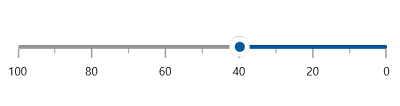## Vertical Slider

The orientation of `slider` can be changed by using the `Orientation` property.

When the `Orientation` property is `Vertical`, the `slider` will be rendered in bottom-to-top vertical direction.

``````<slider:SfSlider Orientation="Vertical"
ShowTicks="True"
ShowLabels="True"
Interval="20"
Value="40" />``````
``````SfSlider sfSlider = new SfSlider();
sfSlider.Orientation = Orientation.Vertical;
sfSlider.ShowTicks = true;
sfSlider.ShowLabels = true;
sfSlider.Interval = 20;
sfSlider.Value = 40;
this.Content = sfSlider;``````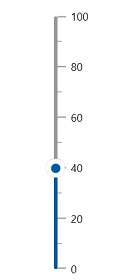## Events

### ValueChanged

The `ValueChanged` event occurs each time when a `Value` gets changed. You can get the following values in this event args:

``````<slider:SfSlider Value="50"
ValueChanged="SfSlider_ValueChanged" />``````
``````private void SfSlider_ValueChanged(object sender, SliderValueChangedEventArgs e)
{
var oldValue = e.OldValue;
var newValue = e.NewValue;
}``````